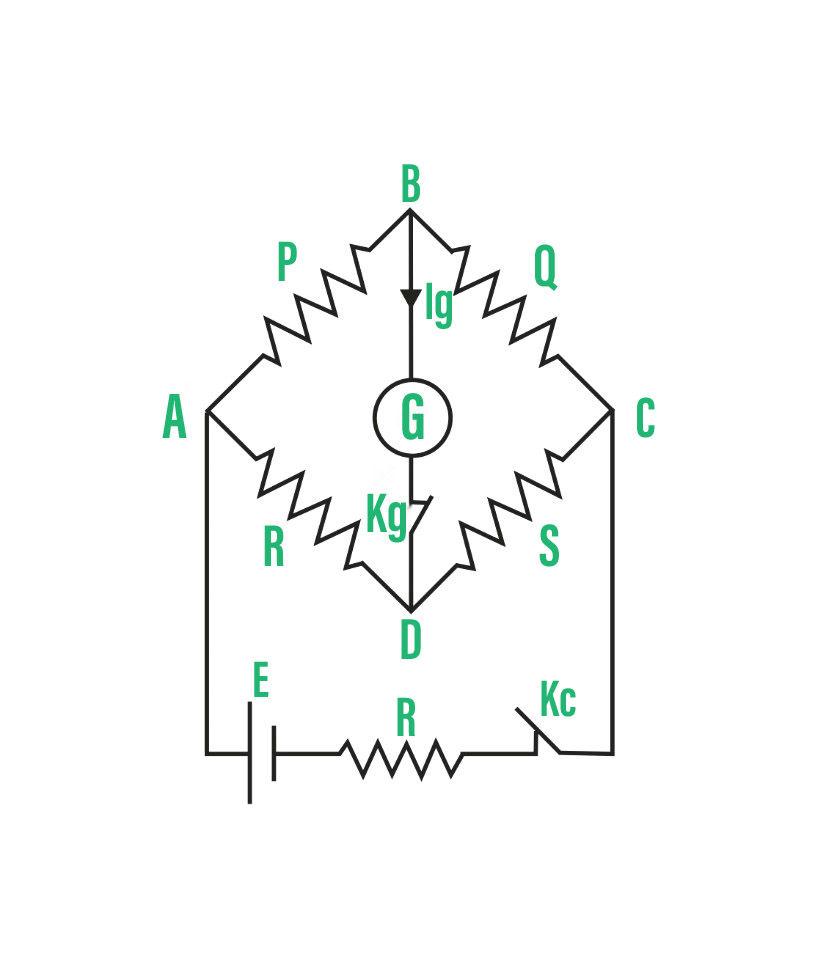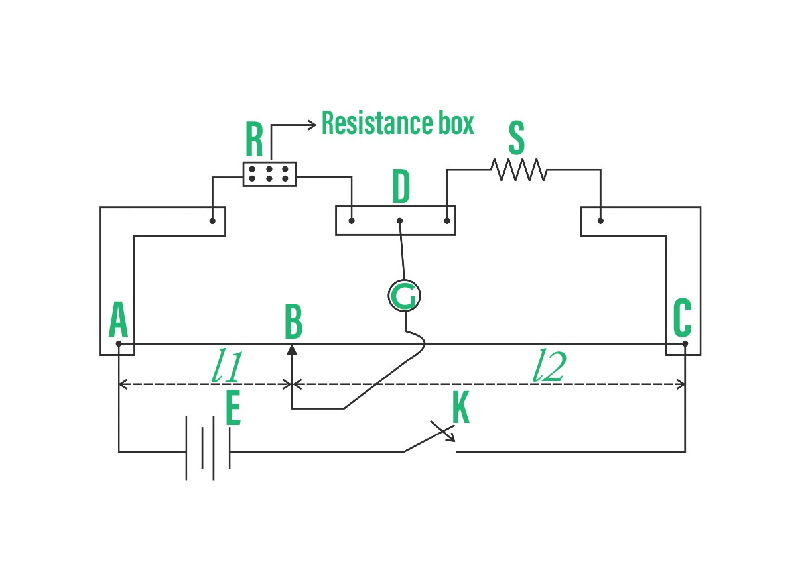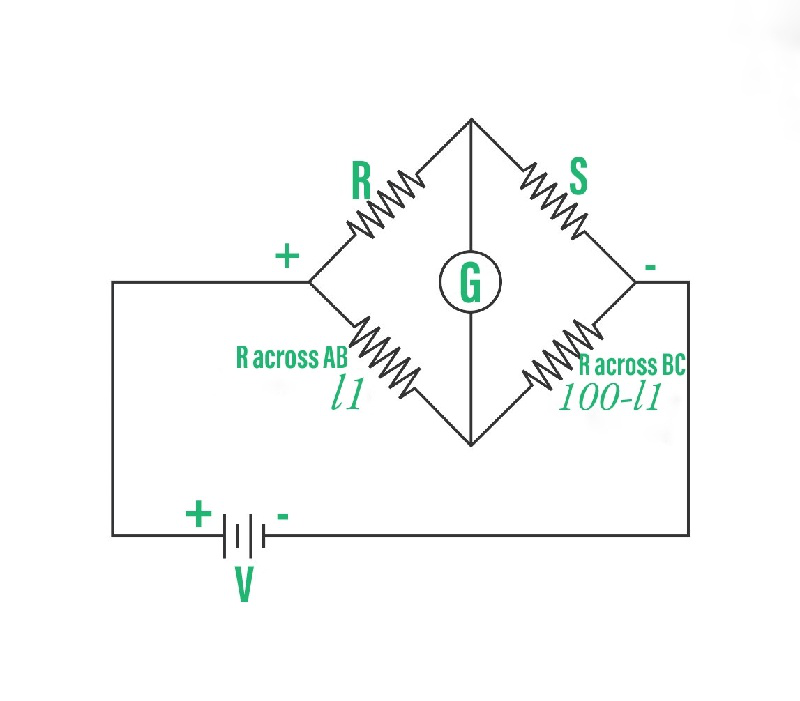Open In App
Related Articles
• CBSE Class 12 Physics Notes

# Meter Bridge – Explanation, Construction, Working, Sample Problems

An electric flow is a flood of charged particles, like electrons or particles, traveling through an electrical conveyor or space. It is estimated as the net pace of stream of electric charge through a surface or into a control volume. The moving particles are called charge transporters, which might be one of a few sorts of particles, contingent upon the conductor. In electric circuits, the charge transporters are regularly electrons traveling through a wire.

In semiconductors, they can be electrons or openings. In an electrolyte, the charge transporters are particles, while in plasma, an ionized gas, they are particles and electrons. The SI unit of electric flow is the ampere, or amp, which is the progression of electric charge across a surface at the pace of one coulomb each second. The ampere is a SI base unit Electric flow is estimated utilizing a gadget called an ammeter.

Electric flows make attractive fields, which are utilized in engines, generators, inductors, and transformers. In normal conductors, they cause Joule warming, which makes light in radiant lights. Time-shifting flows emanate electromagnetic waves, which are utilized in media communications to communicate data.

### Meter Bridge

A meter connect is an electrical contraption utilizing which we can quantify the worth of obscure obstruction. It is made utilizing a meter long wire of uniform cross-area. This wire is either nichrome or manganin or constantan since they offer high opposition and low-temperature coefficient of obstruction. A meter scaffold or Slide wire connect is planned from a Wheatstone connect. It is the most fundamental and useful utilization of a Wheatstone connect.

Principle of a Meter Bridge:

The rule of working of a meter connect is equivalent to the rule of a Wheatstone connect. A Wheatstone connect depends on the rule of invalid avoidance, for example at the point when the proportion of protections in the two arms is equivalent, no current will move through the centre arm of the circuit.

Think about the outline of the Wheatstone connect as displayed underneath. It comprises four obstructions P, Q, R and S with a battery of emf E.Meter Bridge

In the reasonable condition, no current moves through the galvanometer and terminals, B and D are at a similar potential.

This condition emerges when,

P/Q = R/S

Construction of a Meter Bridge:

• A meter connect has a 1m long wire of uniform cross-segment region, which is extended tight.
• Between two metal strips which are twisted at right points, this wire is then painstakingly clasped,
• Inside the hole between the metal stripes, protections are associated. In the principal hole, R, an obstruction confine and the subsequent hole, little resistor wire S is associated.
• The endpoints inside which the wire is clipped are associated with a key through the cell.
• A galvanometer is associated with the metallic directly in the centre of the two holes.
• A rider is associated at the opposite finish of the galvanometer (Here, a rider is a metal bar with a blade-like edge toward one side, slides over the potentiometer wire to make an electrical association). The rider is slid over the meter connect wire till the galvanometer shows zero diversion.Construction of Meter Bridge

Working of a Meter Bridge:

• In any case, move the rider to the endpoints of the wire, i.e. A and C. The diversion of the galvanometer ought to be inverse on the two finishes.
• From side A, begin sliding the rider gradually over the wire and cautiously see where the redirection of the galvanometer comes out to be zero.
• In the event that such a point isn’t acquired, take a stab at different obstructions across the extension by changing the opposition on the variable opposition.
• Slide the rider over the wire and cautiously notice the point on the wire where the redirection of the galvanometer comes out to be zero. This is the invalid point as addressed by point ‘B’ in the graph.
• Get the length of the invalid point utilizing the meter scale appended along the wire. This is the ‘adjusting length’ of the meter connected.
• Leave the distance between focuses An and B alone ‘l1‘.
• Leave the distance between focuses B and C alone ‘l2‘, where l2=100–l1.
• At the point when the galvanometer shows invalid diversion, the meter connects acts like a Wheatstone connects and can be addressed as:### Calculation of an Unknown Resistance Using Meter Bridge:

In case S is the obscure opposition in the above circuit, we can ascertain it’s worth utilizing the meter connect. In reasonable condition,

R / Resistance across length AB = S / Resistance across length BC

We realize that the obstruction r of a wire of length l, space of cross-area An and resistivity ρ is given as,

r= ρl / A

Utilizing this connection, in case of ρ be the resistivity and A be the space of cross-part of the given meter connect wire, then, at that point the obstruction across the length,

AB=ρl1 / A

The resistance across the length,

BC=ρl2 / A

Subbing these qualities in the above connection, we get:

R / ρl1 / A = S / ρl2 / A

or

R / l1 = S / l2

R / l1 = S / 100-l1

Thus, the unknown resistance,

S= (100–l1) R / l1

We can ascertain the particular resistivity of the obscure obstruction by utilizing the equation,

ρ = πd2S / 4L

where d is the width of the wire, S is the obscure opposition (of the wire), and L is the length of the wire.

### Sample Questions

Question 1: What is the end blunder in a meter connect?

End mistake happens when the no sizes of the meter scale don’t agree with the beginning of the wire. It is caused because of the moving of zero scale or the wanderer obstruction in the wire.

Question 2: What is a meter bridge, and what is it utilized for?

A meter bridge is an electrical apparatus that is used to measuring the unknown resistance of a conductor. It consists of a wire of length one meter. Hence it is called a meter bridge.

Question 3: In a meter bridge, there are two unknown resistance R and S. Find the ratio of R and S if the galvanometer shows a null deflection at 20cm from one end?

The null deflection in the galvanometer is obtained at 20cm from one end.

Let, L1=20cm

So, L2=100–20=80cm

Thus, the ratio of unknown resistance will be: R / L1=S / L2

Thus, R / S=1 / 4

Question 4:  A 20Ω resistor is connected in the left gap, and an unknown resistance is joined in the right gap of the meter bridge. Also, the null deflection point is shifted by 40cm when the resistors are interchanged. Find the value of unknown resistance?

In the first case, let the deflection point is taken as L. Let the balance point gets shifted to l by 40cm when the resistors are interchanged.

Thus, L– l=40cm

Also, L+ l=100cm

Solving the above equations, we get:

l=30cm

L=70cm

Let R=20Ω

And unknown resistance be S, thus,

R / S=L / l

R / S=70 / 30

20 / S=7 / 3

∴S=8.57Ω

Question 5: For what reason do we utilize constantan or manganin wire in a meter connect?

Constantan, manganin or nichrome wires give a low-temperature coefficient of obstruction, so they are utilized in a meter bridge.

Question 6: Give the equation to gauge the obscure obstruction for a meter connect

The unknown resistance, S=(100–l1) R / l1, where R is known resistance and l1 is the balancing length of the wire.

Question 7: In a meter bridge with a standard resistance of 15 Ω in the right gap, the ratio of balancing length is 3:2. Find the value of the other resistance.

Q=15 Ω , l1:l2 = 3:2

l1/l2 = 3/2

P/Q = l1/l2

P = Q x l1/l2

P= 22.5 Ω

Question 8: In a meter bridge, the value of resistance in the resistance box is 10 Ω. The balancing length is l1 = 55 cm. Find the value of unknown resistance.